## 【Day01】938.二叉搜索树的范围和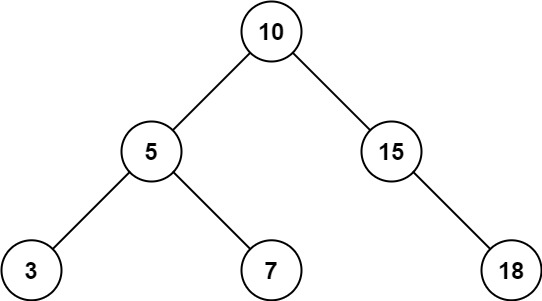输入：root = [10,5,15,3,7,null,18], low = 7, high = 15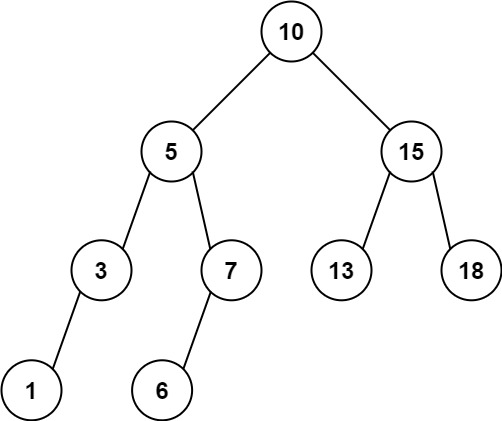输入：root = [10,5,15,3,7,13,18,1,null,6], low = 6, high = 10

• 树中节点数目在范围 [1, 2 * 104] 内
• 1 <= Node.val <= 105
• 1 <= low <= high <= 105
• 所有 Node.val 互不相同

class Solution {
public:
int rangeSumBST(TreeNode* root, int low, int high) {
if (!root) return 0;
int sum = 0;
sum += rangeSumBST(root->left, low, high);
if (root->val >= low && root->val <= high) sum += root->val;
sum += rangeSumBST(root->right, low, high);
return sum;
}
};

class Solution {
public:
int rangeSumBST(TreeNode* root, int low, int high) {
if (!root) return 0;
queue q;
TreeNode* p = root;
int sum = 0;
while (p || q.size()) {
while (p) {
q.push(p);
p = p->left;
}
if (q.size()) {
p = q.front();
q.pop();
if (p->val >= low && p->val <= high) {
sum += p->val;
}
p = p->right;
}
}
return sum;
}
};

## 【Day02】633.平方数之和

输入：c = 5

输入：c = 3

输入：c = 4

输入：c = 2

输入：c = 1

class Solution {
public:
bool judgeSquareSum(int c) {
for (int i = 0; (long long)(i) * i <= c; i++) {
int j = sqrt(c - i * i);
if (i * i + j * j == c)
return true;
}
return false;
}
};

class Solution {
public:
typedef unsigned long long ULL;
bool judgeSquareSum(int c) {
if (c == 0) return true;
unordered_map um;
for (int i = 1; i <= sqrt(c); i++) {
um[i*i]++;
}

for (int i = 1; i <= sqrt(c); i++) {
if (i*i == c || um.count(c - i*i)) return true;
}
return false;
}
};

class Solution {
public:
bool judgeSquareSum(int c) {
int j = sqrt(c);
int i = 0;
while (i <= j) {
if (i*i > c - j*j) {
j--;
} else if (i*i < c - j*j) {
i++;
} else {
return true;
}
}
return false;
}
};

## 【Day03】403.青蛙过河

### 403. 青蛙过河

输入：stones = [0,1,3,5,6,8,12,17]

输入：stones = [0,1,2,3,4,8,9,11]

• 2 <= stones.length <= 2000
• 0 <= stones[i] <= 231 - 1
• stones == 0

class Solution {
public:
unordered_map um;
bool canCross(vector& stones) {

for (int i = 0; i < stones.size(); i++) {
um[stones[i]] = i;  // key:石子值 value是第几块石子
}
if (!um.count(1)) return false;
return dfs(stones, stones.size(), 1, 1);
}
bool dfs(vector& stones, int n, int cur, int k) {
if (cur == n - 1) return true;
for (int i = -1; i <= 1; i++) {
if (k + i == 0) continue;
int next = stones[cur] + k + i;
if (um.count(next)) { // 存在
bool choose = dfs(stones, n, um[next], k + i);
if (choose) return true;
}
}
return false;
}

};

dfs记忆化搜索:

class Solution {
public:
unordered_map um;
vector> memo;
bool canCross(vector& stones) {

for (int i = 0; i < stones.size(); i++) {
um[stones[i]] = i;  // key:石子值 value是第几块石子
}
if (!um.count(1)) return false;
memo = vector>(stones.size(), vector(stones.size(), -1));
return dfs(stones, stones.size(), 1, 1);
}
bool dfs(vector& stones, int n, int cur, int k) {
if (memo[cur][k] != -1) return memo[cur][k];
if (cur == n - 1) return memo[cur][k] = true;
for (int i = -1; i <= 1; i++) {
if (k + i == 0) continue;  // 原地跳过
int next = stones[cur] + k + i;
if (um.count(next)) { // 存在
bool choose = dfs(stones, n, um[next], k + i);
if (choose) return memo[cur][k] = true;
}
}
return memo[cur][k] = false;
}

};

class Solution {
public:
bool canCross(vector& stones) {
int n = stones.size();
if (stones != 1) return false;
vector> dp(n, vector(n));
// dp[i][k] 表示在第i个位置且跳k个单位到第i块石子。
dp = true;
for (int i = 2; i < n; i++) {
for (int j = 1; j < i; j++) {
int k = stones[i] - stones[j];
if (k > j + 1) continue;
// 因为题目要求 青蛙第一次只能跳1步，那么第二次至多跳2步，以此类推，青蛙在第i块（i从0开始）石头上至多只能跳i+1步。在第j块石头上至多只能跳 j+1 步 ，如果k > j+1，说明石头 i 隔石头 j 太远了，远到不满足题目的隐藏规则，所以青蛙必定跳不过去。
dp[i][k] = dp[j][k - 1] || dp[j][k] || dp[j][k+1];
}
}
for (int i = 1; i < n; i++) {
if (dp[n-1][i]) return true;
}
return false;
}
};

class Solution {
public:
bool canCross(vector& stones) {
// dp[i][j] 表示 第 i 个石头是否可以跳 j 步
int n = stones.size();
vector> dp(n, vector(n));
dp = true;
for (int i = 1; i < n; i++) {
bool flag = false;
for (int j = i - 1; j >= 0; j--) {
int k = stones[i] - stones[j];
if (k > i) break;
if (dp[j][k]) {
dp[i][k - 1] = dp[i][k] = dp[i][k + 1] = true;
flag = true;
}
}
if (i == n - 1 && !flag) {
return false;
}
}
return true;
}
};

## 【Day04】137.只出现一次的数字II

#### 137. 只出现一次的数字 II

输入：nums = [2,2,3,2]

输入：nums = [0,1,0,1,0,1,99]

• 1 <= nums.length <= 3 * 104
• -231 <= nums[i] <= 231 - 1
• nums 中，除某个元素仅出现 一次 外，其余每个元素都恰出现 三次

if two == 0:
if n == 0:
one = one
if n == 1:
one = ~one
if two == 1:
one = 0

if two == 0:
one = one ^ n
if two == 1:
one = 0

one = one ^ n & ~two

two = two ^ n & ~one

class Solution {
public:
int singleNumber(vector& nums) {
int two = 0, one = 0;
for (auto x: nums) {
one = (one ^ x) & ~two;
two = (two ^ x) & ~one;
}
return one;
}
};

class Solution {
public:
int singleNumber(vector& nums) {
unordered_map um;
for (auto x : nums) {
um[x]++;
}

for (auto x : um) {
if (x.second == 1) return x.first;
}
return -1;
}
};

## 【Day05】690.员工的重要性

#### 690. 员工的重要性

输入：[[1, 5, [2, 3]], [2, 3, []], [3, 3, []]], 1

• 一个员工最多有一个 直系 领导，但是可以有多个 直系 下属
• 员工数量不超过 2000 。

dfs

class Solution {
public:
unordered_map hash;

int getImportance(vector employees, int id) {
for (auto& p: employees) hash[p->id] = p;
return dfs(id);
}

int dfs(int id) {
auto p = hash[id];
int res = p->importance;
for (auto son: p->subordinates)
res += dfs(son);
return res;
}
};


## 【Day06】554.砖墙

#### 554. 砖墙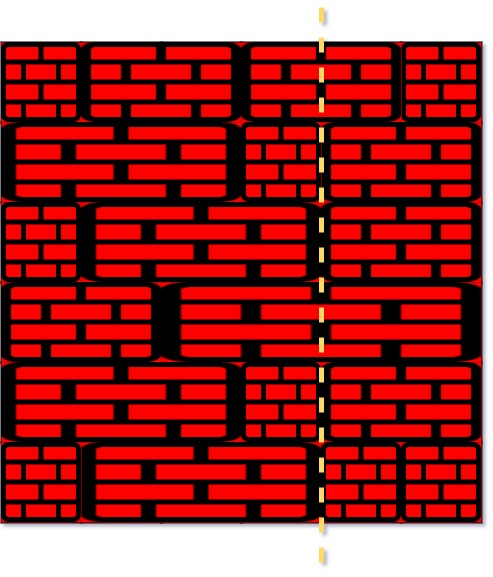输入：wall = [[1,2,2,1],[3,1,2],[1,3,2],[2,4],[3,1,2],[1,3,1,1]]

输入：wall = [,,]

• n == wall.length
• 1 <= n <= 104
• 1 <= wall[i].length <= 104
• 1 <= sum(wall[i].length) <= 2 * 104
• 对于每一行 i ，sum(wall[i]) 应当是相同的
• 1 <= wall[i][j] <= 231 - 1

class Solution {
public:
int leastBricks(vector>& wall) {
unordered_map cnt;
for (auto& line: wall) {
for (int i = 0, s = 0; i + 1 < line.size(); i ++ ) {
s += line[i];
cnt[s] ++ ;
}
}
int res = 0;
for (auto [k, v]: cnt) res = max(res, v);
return wall.size() - res;
}
};


## 【Day07】7.整数反转7. 整数反转

输入：x = 123

输入：x = -123

输入：x = 120

输入：x = 0

• -231 <= x <= 231 - 1

(循环) O(logn)

• 因为int型整数逆序后可能会溢出，所以我们要用long long记录中间结果；

• 在C++中，负数的取模运算和数学意义上的取模运算不同，结果还是负数，比如 −12%10=−2−12%10=−2，所以我们不需要对负数进行额外处理。

class Solution {
public:
int reverse(int x) {
int res = 0;
while (x) {
if (x > 0 && res > (INT_MAX - x % 10) / 10) return 0;
if (x < 0 && res < (INT_MIN - x % 10) / 10) return 0;
res = res * 10 + x % 10;
x /= 10;
}
return res;
}
};


## 【Day08】1473.粉刷房子III

#### 1473. 粉刷房子 III

• houses[i]：是第 i 个房子的颜色，0 表示这个房子还没有被涂色。
• cost[i][j]：是将第 i 个房子涂成颜色 j+1 的花费。

输入：houses = [0,0,0,0,0], cost = [[1,10],[10,1],[10,1],[1,10],[5,1]], m = 5, n = 2, target = 3

输入：houses = [0,2,1,2,0], cost = [[1,10],[10,1],[10,1],[1,10],[5,1]], m = 5, n = 2, target = 3

输入：houses = [0,0,0,0,0], cost = [[1,10],[10,1],[1,10],[10,1],[1,10]], m = 5, n = 2, target = 5

输入：houses = [3,1,2,3], cost = [[1,1,1],[1,1,1],[1,1,1],[1,1,1]], m = 4, n = 3, target = 3

• m == houses.length == cost.length
• n == cost[i].length
• 1 <= m <= 100
• 1 <= n <= 20
• 1 <= target <= m
• 0 <= houses[i] <= n
• 1 <= cost[i][j] <= 10^4

1. 设状态 f(i,j,k) 表示处理了前 i 个房屋，有 j 个社区，最后一个房屋的颜色为 k 的最小花费，其中房屋的有效下标从 1 开始。建立辅助数组 g(i,j,k) 表示同样含义下，最后一个房屋颜色 不是 k 的最小花费。

2. 初始时，f(0,0,k)=g(0,0,k)=0，其余为正无穷或者待定。
转移时，分两种情况

3. 如果第 i 个房屋已经有了颜色 c，则有两种选择，上一个房屋颜色为 c 或者不为 c，转移
$$f(i, j, c)=\min (f(i-1, j, c), g(i-1, j-1, c))$$

4. 如果第 i 个房屋没有颜色，则枚举一个颜色 k，然后同样根据上一个房屋的颜色，转移
$$f(i, j, k)=\min (f(i-1, j, k), g(i-1, j-1, k))+\operatorname{cost}(i, k-1)_{\text {。 }}$$

5. 对于 g 数组的维护如下，假设当前需要维护前 i 个房屋且有 j 个社区下的 g 数组，则我们找 f(i,j,k) 中的最小值 m1 和次小值 m2。如果 m1=m2，则说明对于所有 k， g(i,j,k)=m1；否则，对于 f(i,j,k0)=m1 的那个 k0，其 g(i,j,k0)=m2g，其余 k≠k0都有 g(i,j,k)=m1。

6. 最终答案为
$$\min (f(m, \text { target }, k)$$

class Solution {
public:
int minCost(vector& houses, vector>& cost, int m, int n, int target) {
const int MAX = 0x3f3f3f3f;
const int M = 110;
const int N = 30;

int f[M][M][N], g[M][M][N];
memset(f, 0x3f, sizeof(f));
memset(g, 0x3f, sizeof(g));

for (int k = 1; k <= n; k++)
f[k] = g[k] = 0;

for (int i = 1; i <= m; i++)
for (int j = 1; j <= min(i, target); j++) {
if (houses[i - 1] > 0) {
int c = houses[i - 1];
f[i][j][c] = min(f[i - 1][j][c], g[i - 1][j - 1][c]);
} else {
for (int k = 1; k <= n; k++)
f[i][j][k] = min(f[i - 1][j][k], g[i - 1][j - 1][k])
+ cost[i - 1][k - 1];
}

int m1 = MAX, m2 = MAX;
for (int k = 1; k <= n; k++)
if (m1 > f[i][j][k]) {
m2 = m1;
m1 = f[i][j][k];
} else if (m2 > f[i][j][k])
m2 = f[i][j][k];

if (m1 == m2) {
for (int k = 1; k <= n; k++)
g[i][j][k] = m1;
} else {
for (int k = 1; k <= n; k++)
if (f[i][j][k] == m1) g[i][j][k] = m2;
else g[i][j][k] = m1;
}
}

int ans = MAX;
for (int k = 1; k <= n; k++)
ans = min(ans, f[m][target][k]);

if (ans == MAX)
ans = -1;

return ans;
}
};


## 【Day09】740.删除并获得点数

#### 740. 删除并获得点数](https://leetcode-cn.com/problems/delete-and-earn/)

输入：nums = [3,4,2]

输入：nums = [2,2,3,3,3,4]

• 1 <= nums.length <= 2 * 104
• 1 <= nums[i] <= 104

nums = [2, 2, 3, 3, 3, 4]

all=[0, 0, 2, 3, 1];

$$\mathrm{dp}[\mathrm{i}]=\text { Math.max }(\mathrm{d} \mathrm{p}[\mathrm{i}-1], \mathrm{dp}[\mathrm{i}-2]+\mathrm{nums}[\mathrm{i}])$$

$$\mathrm{dp}[\mathrm{i}]=\text { Math.max }\left(\mathrm{dp}[\mathrm{i}-1], \mathrm{dp}[\mathrm{i}-2]+\mathrm{i}^{\star} \mathrm{all}[\mathrm{i}]\right)$$

class Solution {
public:
int deleteAndEarn(vector& nums) {
if(nums.size() < 1) return 0;
int maxn = 0;
for(int it : nums)
maxn = max(maxn, it);
vector cnt(maxn+1), dp(maxn+1);
for(int it : nums)
cnt[it]++;
dp = cnt;
for(int i = 2; i <= maxn; i++)
dp[i] = max(dp[i-1], dp[i-2] + cnt[i] * i);
return dp[maxn];
}
};

## 【Day10】1720. 解码异或后的数组

#### 1720. 解码异或后的数组

输入：encoded = [1,2,3], first = 1

输入：encoded = [6,2,7,3], first = 4

• 2 <= n <= 104
• encoded.length == n - 1
• 0 <= encoded[i] <= 105
• 0 <= first <= 105

class Solution {
public:
vector decode(vector& encoded, int first) {
int n = encoded.size();
vector arr(n + 1);
arr = first;
for (int i = 0; i < n; i ++)
arr[i + 1] = arr[i] ^ encoded[i];
return arr;
}
};


## 【Day11】1486.数组异或操作

#### 1486. 数组异或操作

输入：n = 5, start = 0

"^" 为按位异或 XOR 运算符。

输入：n = 4, start = 3

输入：n = 1, start = 7

输入：n = 10, start = 5

• 1 <= n <= 1000
• 0 <= start <= 1000
• n == nums.length

class Solution {
public:
int xorOperation(int n, int start)
{
int result = start, i;
for (i = 1; i < n; i++)
{
result = result ^ (start + i * 2);
}
return result;
}
};

## 【Day12】1723.完成所有工作的最短时间

#### 1723. 完成所有工作的最短时间

输入：jobs = [3,2,3], k = 3

输入：jobs = [1,2,4,7,8], k = 2

1 号工人：1、2、8（工作时间 = 1 + 2 + 8 = 11）
2 号工人：4、7（工作时间 = 4 + 7 = 11）

• 1 <= k <= jobs.length <= 12
• 1 <= jobs[i] <= 107

[t1,0,0,0,0]

[0,t1,0,0,0]
...

56号测试用例：

[5,5,4,4,4]
2

class Solution {
public:
int k;
vector  block;
bool backtracking(vector& jobs,int id,int lim) {
if(id==-1) return true; //出口 全部放完了

bool res=false;
int last=INT_MIN;

for(int i=0;i& jobs, int k) {
this->k = k;
int s=0; //区间左端点（最小）
int e=0; //区间右端点（最大）
int m;//中间
int n = jobs.size()-1;//起手下标
block = vector(k,0); // 拷贝初始化
sort(jobs.begin(),jobs.end());
s=jobs[n];//结果最小应是 jobs[i] 的最大值

for(auto i : jobs) { //最大应是 sum(jobs.begin(),jobs.end()) 小心越界
if(e<=INT_MAX-i) e+=i;
else e=INT_MAX;
}
while(s!=e) {
int m = (s+e)/2;
if(backtracking(jobs,n,m)) { //成功 尝试缩减区间
e=m;//m无法排除
}else {//失败 扩大
s=m+1;//m肯定不对最少加1
}
}
return s;
}
};

## 【Day13】1482.制作m束花所需的最少天数

#### 1482. 制作 m 束花所需的最少天数

输入：bloomDay = [1,10,3,10,2], m = 3, k = 1

1 天后：[x, _, _, _, _]   // 只能制作 1 束花
2 天后：[x, _, _, _, x]   // 只能制作 2 束花
3 天后：[x, _, x, _, x]   // 可以制作 3 束花，答案为 3

输入：bloomDay = [1,10,3,10,2], m = 3, k = 2

输入：bloomDay = [7,7,7,7,12,7,7], m = 2, k = 3

7 天后：[x, x, x, x, _, x, x]

12 天后：[x, x, x, x, x, x, x]

输入：bloomDay = [1000000000,1000000000], m = 1, k = 1

输入：bloomDay = [1,10,2,9,3,8,4,7,5,6], m = 4, k = 2

• bloomDay.length == n
• 1 <= n <= 10^5
• 1 <= bloomDay[i] <= 10^9
• 1 <= m <= 10^6
• 1 <= k <= n

• [0, x)天无法制作出来m束
• [x,maxDay]可以制作出m束

class Solution {
public:
int minDays(vector& bloomDay, int m, int k) {
int n = bloomDay.size();
if (m * k  > n) return -1;
int l = 0, r = 0;
for (auto x : bloomDay) {
r = max(r, x);
}
while(l < r) {
int mid = l + r >> 1;
if (check(bloomDay, mid, m, k)) {
r = mid;
} else {
l = mid + 1;
}
}
return l;
}
bool check(vector& bloomDay, int mid, int m, int k) {
int sum = 0;
// 计算是否满足m束花且每一束都有相邻的k个
for (int i = 0; i < bloomDay.size() && sum < m; i++) {
int cur_sum = 0;
if (bloomDay[i] <= mid) { // 可以开
cur_sum++;
int j = i + 1;
while (j < bloomDay.size() && bloomDay[j] <= mid && cur_sum < k) {
j++;
cur_sum++;
}
if (cur_sum == k) sum++;
i = j - 1;
}
}
return sum >= m;
}
};

## 【Day14】872.叶子相似的树

#### 872. 叶子相似的树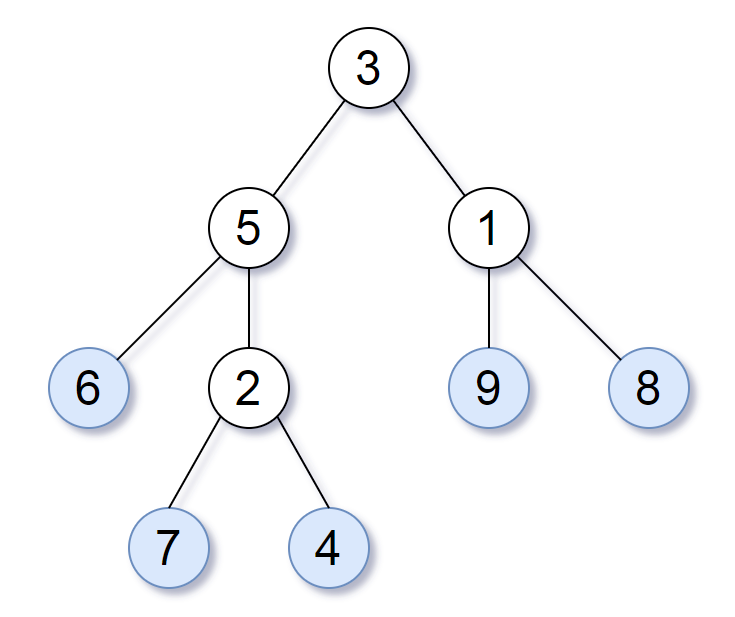输入：root1 = [3,5,1,6,2,9,8,null,null,7,4], root2 = [3,5,1,6,7,4,2,null,null,null,null,null,null,9,8]

输入：root1 = , root2 = 

输入：root1 = , root2 = 

输入：root1 = [1,2], root2 = [2,2]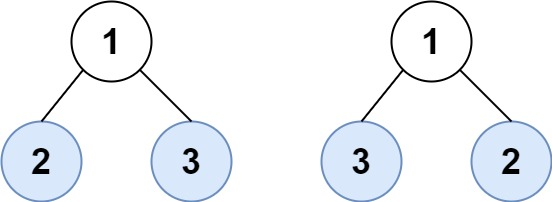输入：root1 = [1,2,3], root2 = [1,3,2]

• 给定的两棵树可能会有 1 到 200 个结点。
• 给定的两棵树上的值介于 0 到 200 之间。

class Solution {
public:
int index = 0;
bool leafSimilar(TreeNode* root1, TreeNode* root2) {
vector r1;
dfs1(root1, r1);
return dfs2(root2, r1) && index == r1.size();
}
void dfs1(TreeNode* root, vector& res) {
if (!root) return;
if (!root->left && !root->right) {
res.push_back(root->val);
return;
}
dfs1(root->left, res);
dfs1(root->right, res);
}
bool dfs2(TreeNode* root, vector& res) {
if (!root) return true;
if (!root->left && !root->right) {
if (index >= res.size()) return false;
if (root->val != res[index]) return false;
index++;
return true;
}
return dfs2(root->left, res) && dfs2(root->right, res);
}

};

## 【Day15】1734.解码异或后的排列

#### 1734. 解码异或后的排列

输入：encoded = [3,1]

输入：encoded = [6,5,4,6]

• 3 <= n < 105
• n 是奇数。
• encoded.length == n - 1

perm = [A,B,C,D,E]

encoded = [A^B, B^C, C^D, D^E]

A^B^C^D^E = total

total ^ (B^C^D^E) = first

class Solution {
public:
vector decode(vector& encoded) {
int n = encoded.size() + 1;
int total = 0;
for (int i = 1; i <= n; i++) {
total ^= i;
}

int e = 0;
for (int i = 1; i < n - 1; i += 2) {
e ^= encoded[i];
}

vector ans(n);
ans = total ^ e;
for (int i = 1; i < n; i++) {
ans[i] = ans[i-1] ^ encoded[i-1];
}
return ans;
}
};

## 【Day16】1310.子数组异或查询

#### 1310. 子数组异或查询

输入：arr = [1,3,4,8], queries = [[0,1],[1,2],[0,3],[3,3]]

1 = 0001
3 = 0011
4 = 0100
8 = 1000

[0,1] = 1 xor 3 = 2
[1,2] = 3 xor 4 = 7
[0,3] = 1 xor 3 xor 4 xor 8 = 14
[3,3] = 8

输入：arr = [4,8,2,10], queries = [[2,3],[1,3],[0,0],[0,3]]

• 1 <= arr.length <= 3 * 10^4
• 1 <= arr[i] <= 10^9
• 1 <= queries.length <= 3 * 10^4
• queries[i].length == 2
• 0 <= queries[i] <= queries[i] < arr.length

class Solution {
public:
vector xorQueries(vector& arr, vector>& queries) {
vector ans;
int sum[arr.size() + 1];
for (int i = 1; i <= arr.size(); i++) {
sum[i] = sum[i - 1] ^ arr[i - 1];
}

for (auto q : queries) {
ans.push_back(sum[q + 1] ^ sum[q]);
}
return ans;
}

## 【Day17】1269.停在原地的方案数

#### 1269. 停在原地的方案数

输入：steps = 3, arrLen = 2

输入：steps = 2, arrLen = 4

输入：steps = 4, arrLen = 2

1 <= steps <= 500
1 <= arrLen <= 10^6

pos表示移动的index,st表示当前还剩步数。结果超时- _ -

class Solution {
public:
const int N = 1000000007;
int numWays(int steps, int arrLen) {
int res = dfs(steps, arrLen, 0);
return res % N;
}

int dfs(int st, int arrLen, int pos) {
if (st < 0 || pos < 0 || pos >= arrLen) return 0;
if (st == 0 && pos == 0) {
return 1;
}
int ans = 0;
if (pos >= 0 && pos <= arrLen - 1)
// 不动
ans = dfs(st - 1, arrLen, pos);
if (pos >= 1)
// 向左
ans += dfs(st - 1, arrLen, pos - 1);
if (pos <= arrLen - 2)
// 向右
ans += dfs(st- 1, arrLen, pos + 1);
return ans;
}
};

int memo;
// vector> memo;
class Solution {
public:
static const int N = 1000000007;
int numWays(int steps, int arrLen) {
memset(memo, -1, sizeof(memo));
//   memo = vector>(505, vector(505,-1));
int res = dfs(steps, arrLen, 0);
return res % N;
}

int dfs(int st, int arrLen, int pos) {
if (st < 0 || pos < 0 || pos >= arrLen) return 0;
if (memo[st][pos] != -1) return memo[st][pos];
if (st == 0 && pos == 0) {
return memo[st][pos] = 1;
}
int ans = 0;
if (pos >= 0 && pos <= arrLen - 1)
// 不动
ans = dfs(st - 1, arrLen, pos) % N;
if (pos >= 1)
// 向左
ans =  ans % N + dfs(st - 1, arrLen, pos - 1) % N;
if (pos <= arrLen - 2)
// 向右
ans = ans % N + dfs(st- 1, arrLen, pos + 1) % N;
return memo[st][pos] = ans;
}
};

class Solution {
public:
static const int N = 1000000007;
int numWays(int steps, int arrLen) {
int maxLen = min(steps, arrLen);
long long dp[steps + 1][maxLen + 1];
memset(dp, 0, sizeof dp);
dp = 1;
dp = 1; // step=1 pos=1

for (int s = 1; s <= steps; s++) {
for (int l = 0; l < maxLen; l++) {
// 原地
dp[s][l] = dp[s][l] + dp[s - 1][l];
// 右
dp[s][l] = dp[s][l]  + dp[s - 1][l + 1];
// 左

if (l - 1 >= 0)
dp[s][l] =  dp[s][l] + dp[s - 1][l - 1];

dp[s][l] %= N;
}
}
return dp[steps];
}
};

## 【Day18】12.整数转罗马数字

#### 12. 整数转罗马数字

字符          数值
I             1
V             5
X             10
L             50
C             100
D             500
M             1000

• I 可以放在 V (5) 和 X (10) 的左边，来表示 4 和 9。
• X 可以放在 L (50) 和 C (100) 的左边，来表示 40 和 90。
• C 可以放在 D (500) 和 M (1000) 的左边，来表示 400 和 900。

输入: num = 3

输入: num = 4

输入: num = 9

输入: num = 58

输入: num = 1994

• 1 <= num <= 3999

class Solution {
public:
string intToRoman(int num) {
int values[] = {1000, 900, 500, 400, 100, 90, 50, 40, 10, 9, 5, 4, 1};
string reps[] = {"M", "CM", "D", "CD", "C", "XC", "L", "XL", "X", "IX", "V", "IV", "I"};

string ans;
int
for (int i = 0; i < 13; i ++ )
while(num >= values[i])
{
num -= values[i];
ans += reps[i];
}
return ans;
}
};

## 【Day19】13.罗马数字转整数

#### 13. 罗马数字转整数

字符          数值
I             1
V             5
X             10
L             50
C             100
D             500
M             1000

• I 可以放在 V (5) 和 X (10) 的左边，来表示 4 和 9。
• X 可以放在 L (50) 和 C (100) 的左边，来表示 40 和 90。
• C 可以放在 D (500) 和 M (1000) 的左边，来表示 400 和 900。

输入: "III"

输入: "IV"

输入: "IX"

输入: "LVIII"

输入: "MCMXCIV"

• 1 <= s.length <= 15
• s 仅含字符 (‘I’, ‘V’, ‘X’, ‘L’, ‘C’, ‘D’, ‘M’)
• 题目数据保证 s 是一个有效的罗马数字，且表示整数在范围 [1, 3999] 内
• 题目所给测试用例皆符合罗马数字书写规则，不会出现跨位等情况。
• IL 和 IM 这样的例子并不符合题目要求，49 应该写作 XLIX，999 应该写作 CMXCIX 。
• 关于罗马数字的详尽书写规则，可以参考 罗马数字 - Mathematics 。

• 当小值在大值的左边，则减小值，如 IV=5-1=4；

• 当小值在大值的右边，则加小值，如 VI=5+1=6；

• 小值放在大值的左边，就是做减法，否则为加法。

class Solution {
public:
int romanToInt(string s) {
int r=0;

for(int i=0;i

## 【Day20】421.数组中两个数的最大异或值

#### 421. 数组中两个数的最大异或值

输入：nums = [3,10,5,25,2,8]

输入：nums = 

输入：nums = [2,4]

输入：nums = [8,10,2]

输入：nums = [14,70,53,83,49,91,36,80,92,51,66,70]

• 1 <= nums.length <= 2 * 104
• 0 <= nums[i] <= 231 - 1

struct Node{
Node* next = {nullptr};
};
class Solution {
public:
void insert(int num, Node* root) {
for (int i = 30; i >= 0; --i) {
int t = (num >> i & 1);
if (!root->next[t]) {
root->next[t] = new Node();
}
root = root->next[t];
}
}
int findMaximumXOR(vector& nums) {
Node* root = new Node();
for (auto val : nums) {
insert(val, root);
}
int res = 0, tmp = 0;
Node* p = root;
for (auto val : nums) {
p = root; tmp = 0;
for (int i = 30; i >= 0; --i) {
int t = (val >> i) & 1;
if (p->next[!t]) {
p = p->next[!t];
tmp += (1 << i);
}else p = p->next[t];
}
res = max(res, tmp);
}
return res;
}
};

class Solution {
public:
int findMaximumXOR(vector& nums) {
sort(nums.begin(), nums.end(), [](auto a, auto b) {return a > b;});
int ans = 0;
for (int i = 0; i < nums.size() - 1; i++) {
for (int j = i + 1; j < nums.size(); j++) {
long long x = (long long)nums[i] + nums[j];
if (ans > x) break;
ans = max(ans, nums[i] ^ nums[j]);
}
}
return ans;
}
};

## 【Day21】993.二叉树的堂兄弟节点

#### 993. 二叉树的堂兄弟节点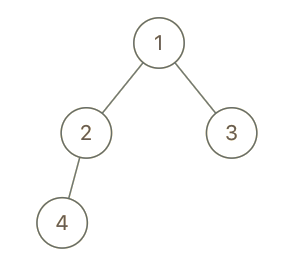输入：root = [1,2,3,4], x = 4, y = 3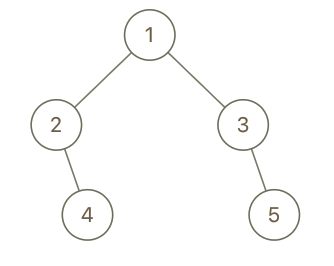输入：root = [1,2,3,null,4,null,5], x = 5, y = 4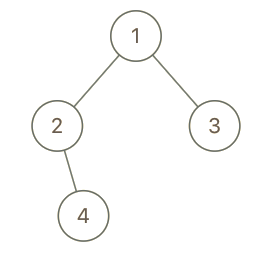输入：root = [1,2,3,null,4], x = 2, y = 3

• 二叉树的节点数介于 2 到 100 之间。
• 每个节点的值都是唯一的、范围为 1 到 100 的整数。

class Solution {
public:
bool isCousins(TreeNode* root, int x, int y) {
queue q;
q.push(root);
int cnt = 1;
while (q.size()) {
int sz = q.size();
bool xt = false, yt = false;
int xcnt, ycnt;
while (sz--) {
auto p = q.front();
q.pop();
if (p) {
if (x == p->val) {
xt = true;
xcnt = cnt;
}
if (y == p->val) {
yt = true;
ycnt = cnt;
}

if (xt && yt && int(xcnt / 2) != int(ycnt / 2) ) {
return true;
}
q.push(p->left); q.push(p->right);
}
cnt++;
}
}
return false;
}
};

## 【Day22】1442.形成两个异或相等数组的三元组数目

#### 1442. 形成两个异或相等数组的三元组数目

a 和 b 定义如下：

• a = arr[i] ^ arr[i + 1] ^ … ^ arr[j - 1]
• b = arr[j] ^ arr[j + 1] ^ … ^ arr[k]

输入：arr = [2,3,1,6,7]

输入：arr = [1,1,1,1,1]

输入：arr = [2,3]

输入：arr = [1,3,5,7,9]

输入：arr = [7,11,12,9,5,2,7,17,22]

• 1 <= arr.length <= 300
• 1 <= arr[i] <= 10^8

∵ a = arr[i] ^ arr[i + 1] ^ … ^ arr[j - 1]

​ b = arr[j] ^ arr[j + 1] ^ … ^ arr[k]

∴ arr[i] ^ arr[i + 1] ^ … ^ arr[k] = a ^ b = 0;

a^b=0得到区间[i,k]中有k-i个元组，全部累加即可。区间内三元组的个数为 k - i（因为区间内的任意一个j，都和i，k组成满足题目的一个三元组）。

class Solution {
public:
int countTriplets(vector& arr) {
int ans = 0;
for (int i = 0; i < arr.size(); i++) {
int s = arr[i];
for (int k = i + 1; k < arr.size(); k++) {
s ^= arr[k];
if (s == 0) ans += k - i;
}
}
return ans;
}
};

## 【Day23】1738.找出第 K 大的异或坐标值

#### 1738. 找出第 K 大的异或坐标值

输入：matrix = [[5,2],[1,6]], k = 1

输入：matrix = [[5,2],[1,6]], k = 2

输入：matrix = [[5,2],[1,6]], k = 3

输入：matrix = [[5,2],[1,6]], k = 4

• m == matrix.length
• n == matrix[i].length
• 1 <= m, n <= 1000
• 0 <= matrix[i][j] <= 106
• 1 <= k <= m * n

class Solution {
public:
int prefix;
int kthLargestValue(vector>& matrix, int k) {
int m = matrix.size();
int n = matrix.size();
prefix = matrix;
// 处理第一行
for (int i = 1; i < n; i++) {
prefix[i] = prefix[i - 1] ^ matrix[i];
}
// 处理第一列
for (int i = 1; i < m; i++) {
prefix[i] = prefix[i - 1] ^ matrix[i];
}

for (int i = 1; i < m; i++) {
for (int j = 1; j < n; j++) {
prefix[i][j] = prefix[i - 1][j] ^ prefix[i][j - 1] ^ matrix[i][j] ^ prefix[i - 1][j - 1];
}
}

priority_queue, std::greater> pq;
for (int i = 0; i < m; i++) {
for (int j = 0; j < n; j++) {
pq.push(prefix[i][j]);
if (pq.size() > k) pq.pop();
}
}
return pq.top();
}
};

class Solution {
public:
int prefix;
int m, n;
int kthLargestValue(vector>& matrix, int k) {
m = matrix.size();
n = matrix.size();
prefix = matrix;
// 处理第一行
for (int i = 1; i < n; i++) {
prefix[i] = prefix[i - 1] ^ matrix[i];
}
// 处理第一列
for (int i = 1; i < m; i++) {
prefix[i] = prefix[i - 1] ^ matrix[i];
}

for (int i = 1; i < m; i++) {
for (int j = 1; j < n; j++) {
prefix[i][j] = prefix[i - 1][j] ^ prefix[i][j - 1] ^ matrix[i][j] ^ prefix[i - 1][j - 1];
}
}

int left = 0, right = 1e6;
while (left < right) {
int mid = right - (right - left - 1) / 2;
if (count(mid) < k) { // 缩小值
right = mid - 1;
} else {
left = mid;
}
}
return left;
}
int count (int mid) {
int cnt = 0;
for (int i = 0; i < m; i++) {
for (int j = 0; j < n; j++) {
if (prefix[i][j] >= mid) cnt++;
}
}
return cnt;
}
};

## 【Day24】692.前K个高频单词

#### 692. 前K个高频单词

输入: ["i", "love", "leetcode", "i", "love", "coding"], k = 2

注意，按字母顺序 "i" 在 "love" 之前。

输入: ["the", "day", "is", "sunny", "the", "the", "the", "sunny", "is", "is"], k = 4

出现次数依次为 4, 3, 2 和 1 次。

• 假定 k 总为有效值， 1 ≤ k ≤ 集合元素数。
• 输入的单词均由小写字母组成。

struct Cmp {
bool operator()(const pair& p1, const pair& p2) {
if(p1.second != p2.second) return p1.second > p2.second;
else return p1.first < p2.first;
}
};

class Solution {
public:
vector topKFrequent(vector& words, int k) {
unordered_map m;
for(string& word : words) m[word]++;
vector> sorted_list(m.begin(), m.end());
sort(sorted_list.begin(), sorted_list.end(), Cmp());
vector res;
for(int i = 0; i < k; i++) res.push_back(sorted_list[i].first);
return res;
}
};

## 【Day25】1035.不相交的线

#### 1035. 不相交的线

• nums1[i] == nums2[j]
• 且绘制的直线不与任何其他连线（非水平线）相交。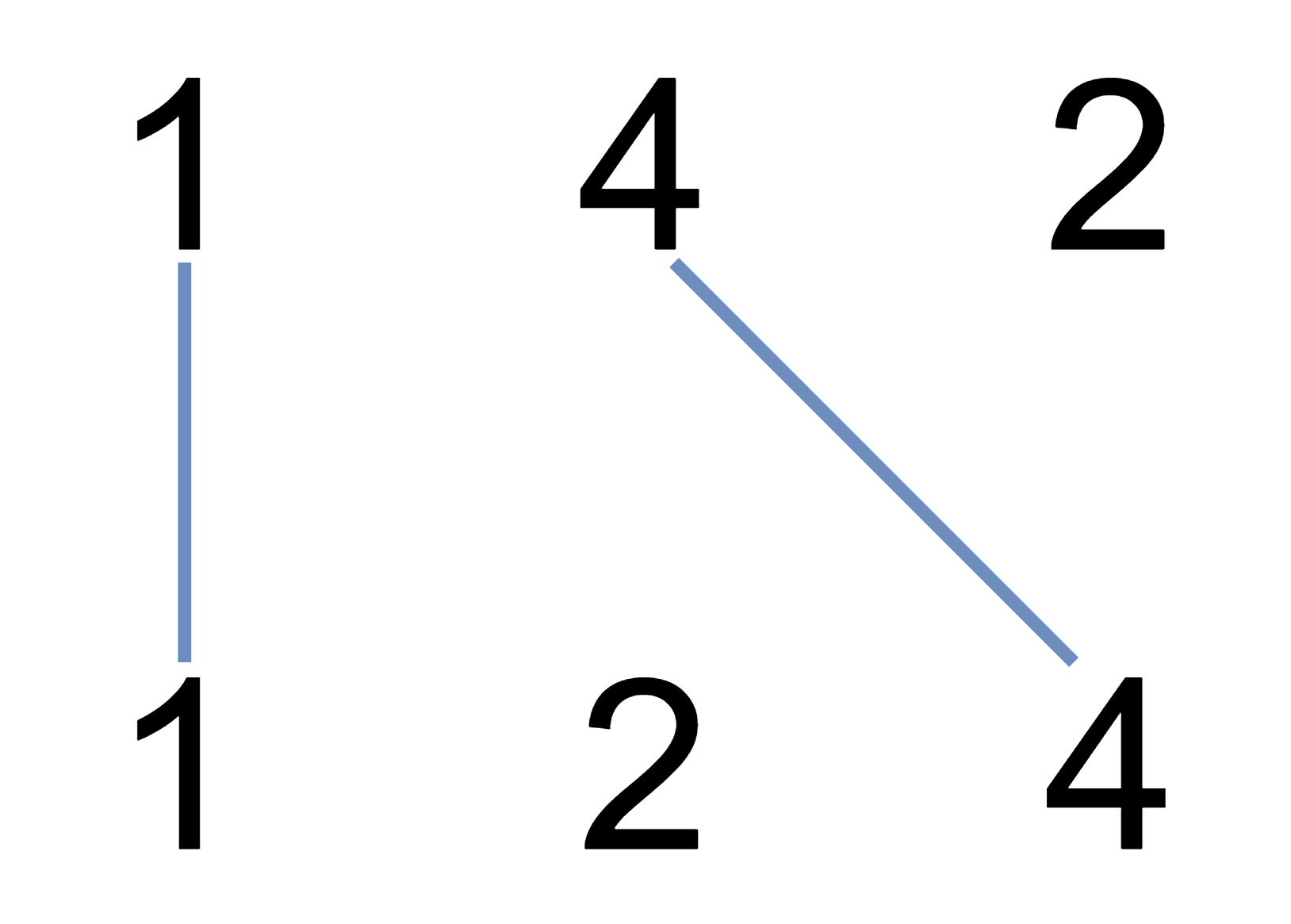输入：nums1 = [1,4,2], nums2 = [1,2,4]

输入：nums1 = [2,5,1,2,5], nums2 = [10,5,2,1,5,2]

输入：nums1 = [1,3,7,1,7,5], nums2 = [1,9,2,5,1]

• 1 <= nums1.length <= 500
• 1 <= nums2.length <= 500
• 1 <= nums1[i], nums2[i] <= 2000

class Solution {
public:
vector> dp;
int maxUncrossedLines(vector& nums1, vector& nums2) {
int n = nums1.size(), m = nums2.size();
dp = vector>(n + 1, vector(m + 1, 0));
for (int i = 1; i <= n; i++) {
for (int j = 1; j <= m; j++) {
if (nums1[i - 1] == nums2[j - 1]) dp[i][j] = dp[i - 1][j - 1] + 1;
else dp[i][j] = max(dp[i-1][j], dp[i][j-1]);
}
}
return dp[n][m];
}
};

## 【Day26】810.黑板异或游戏

#### 810. 黑板异或游戏

输入: nums = [1, 1, 2]

Alice 有两个选择: 擦掉数字 1 或 2。

• 1 <= N <= 1000
• 0 <= nums[i] <= 2^16

class Solution {
public:
bool xorGame(vector& nums) {
//数组元素个数是奇数/偶数，有决定性作用：
//如果是偶数，先手必胜；
//如果是奇数，只有当一上来所有元素异或的结果为0，先手才获胜，
//否则，接下来轮到后手，此时元素个数为偶数，则后手必胜，先手必败！
int len = nums.size(), t = 0;
if(len % 2)
{
for(auto& x:nums) t ^= x; //所有元素异或的结果
if(t) return false;
else return true;
}
else return true;
}
};

## 【Day27】1707.与数组中元素的最大异或值

#### 1707. 与数组中元素的最大异或值

输入：nums = [0,1,2,3,4], queries = [[3,1],[1,3],[5,6]]

1) 0 和 1 是仅有的两个不超过 1 的整数。0 XOR 3 = 3 而 1 XOR 3 = 2 。二者中的更大值是 3 。
2) 1 XOR 2 = 3.
3) 5 XOR 2 = 7.

输入：nums = [5,2,4,6,6,3], queries = [[12,4],[8,1],[6,3]]

• 1 <= nums.length, queries.length <= 105
• queries[i].length == 2
• 0 <= nums[j], xi, mi <= 109

const int N = 1e5 + 50, M = 32 * N;
int son[M];
int idx;

void insert(int x){
int p = 0;
for(int i = 31; i >= 0; i--){
int u = (x >> i) & 1;
if(!son[p][u]) son[p][u] = ++idx;
p = son[p][u];
}
}
int query(int x){
int ans = 0, p = 0;
for(int i = 31; i >= 0; i--){
int u = (x >> i) & 1;
if(son[p][!u]) {
ans += (1 << i);
p = son[p][!u];
}
else p = son[p][u];
}
return ans;
}

class Solution {
public:
vector maximizeXor(vector& nums, vector>& queries) {
memset(son,0,sizeof son);
idx = 0;
sort(nums.begin(),nums.end());
//离线思想，因此需要对queries加一个pos，因为回答是乱序的
int pos = 0;
for(auto& q: queries){
q.push_back(pos++);
}
sort(queries.begin(),queries.end(),[](const auto& a,const auto& b){
return a < b;
});
vector ans(queries.size());
int cur = 0;
for(const auto& q : queries){
int xi = q, mi = q,id = q;
while(cur < nums.size() and nums[cur] <= mi){
insert(nums[cur]);
cur++;
}
if(cur == 0) ans[id] = -1;
else ans[id] = query(xi);
}
return ans;
}
};

## 【Day28】664.奇怪的打印机

#### 664. 奇怪的打印机

• 打印机每次只能打印由 同一个字符 组成的序列。
• 每次可以在任意起始和结束位置打印新字符，并且会覆盖掉原来已有的字符。

输入：s = "aaabbb"

输入：s = "aba"

• 1 <= s.length <= 100
• s 由小写英文字母组成

dp[i][j] = min(dp[i][j], dp[i][k] + dp[k+1][j])

class Solution {
public:
int strangePrinter(string s) {
// aba
// aaabbb
int n = s.size();
int dp[n][n];
memset(dp, 0x3f3f3f3f, sizeof(dp));
for (int i = 0; i < n; i++) {
dp[i][i] = 1;
}

for (int i = n - 1; i >= 0; i--) {
for (int j = i + 1; j < n; j++) {
if (s[i] == s[j]) {
dp[i][j] = dp[i][j - 1];
} else {
for (int k = i; k < j; k++) {
dp[i][j] = min(dp[i][j], dp[i][k] + dp[k + 1][j]);
}
}
}
}
return dp[n - 1];
}
};

class Solution {
public:
int strangePrinter(string s) {
// aba
// aaabbb
int n = s.size();
int dp[n][n];
memset(dp, 0x3f3f3f3f, sizeof(dp));
for (int i = 0; i < n; i++) {
dp[i][i] = 1;
}

for (int l = 2; l <= n; l++) {
for (int i = 0; i < n - l + 1; i++) {
int j = l + i - 1;
if (s[i] == s[j]) {
dp[i][j] = dp[i][j - 1];
} else {
for (int k = i; k < j; k++) {
dp[i][j] = min(dp[i][j], dp[i][k] + dp[k + 1][j]);
}
}
}
}

return dp[n - 1];
}
};

## 【Day29】1787.使所有区间的异或结果为零

#### 1787. 使所有区间的异或结果为零

输入：nums = [1,2,0,3,0], k = 1

输入：nums = [3,4,5,2,1,7,3,4,7], k = 3

输入：nums = [1,2,4,1,2,5,1,2,6], k = 3

• 1 <= k <= nums.length <= 2000

• 0 <= nums[i] < 210

题解：

class Solution {
public:
// 1.某一列用了一个全新的数
// 2.每一列用了原来的数

const int N = 1024, INF = 1e8;
int s; // 求众数
int minChanges(vector& nums, int k) {
int n = nums.size(), m = (n + k - 1) / k;
vector> f(k + 1, vector(N, INF));
int cnt = 0, minv = INF; // 每一列代价
// f[i][j] 第i列的异或和为j
f = 0;
for (int i = 1; i <= k; i++) {
int len = m;
memset(s, 0 , sizeof s);
if (n % k && n % k < i) len--;
for (int j = 0; j < len; j ++) {
s[nums[j * k + i - 1]]++;
}
int maxv = 0;
for (int j = 0; j < N; j++) {
maxv = max(maxv, s[j]);
}
cnt += len - maxv;
minv = min(minv, maxv); // 众数最少的那一列  不用众数  而用全新的数

for (int j = 0; j < N; j++) { // 异或和为j
for (int u = 0; u < len; u++) { // 每一行
int x = nums[u * k + i - 1], cost = len - s[x];
f[i][j] = min(f[i][j], f[i - 1][j ^ x] + cost);
}
}
}
// cnt: 每一列的代价
// minv表示 某一列不用众数时的代价 si - maxv -> si 变成全新的数代价
return min(cnt + minv, f[k]);
}
};

## 【Day30】1190.反转每对括号间的子串

#### 1190. 反转每对括号间的子串

输入：s = "(abcd)"

输入：s = "(u(love)i)"

输入：s = "(ed(et(oc))el)"

输入：s = "a(bcdefghijkl(mno)p)q"

• 0 <= s.length <= 2000
• s 中只有小写英文字母和括号
• 我们确保所有括号都是成对出现的class Solution {
public:
string reverseParentheses(string s) {
stack st;
for (auto c : s) {
if (c != ')') {
st.push(c);
} else {
string tmp;
while (st.top() != '(') {
tmp += st.top();
st.pop();
}
st.pop();
for (auto s : tmp) {
st.push(s);
}
}
}
string ans;
while (!st.empty()) {
ans = st.top() + ans; st.pop();
}
return ans;
}
};


转载规则

《暑假LeetCode刷题集合（上）》 Tyzhao 采用 知识共享署名 4.0 国际许可协议 进行许可。
上一篇使用Java开发一个记事本

2021-07-17《X86汇编语言从实模式到保护模式》阅读笔记

2021-05-16
目录